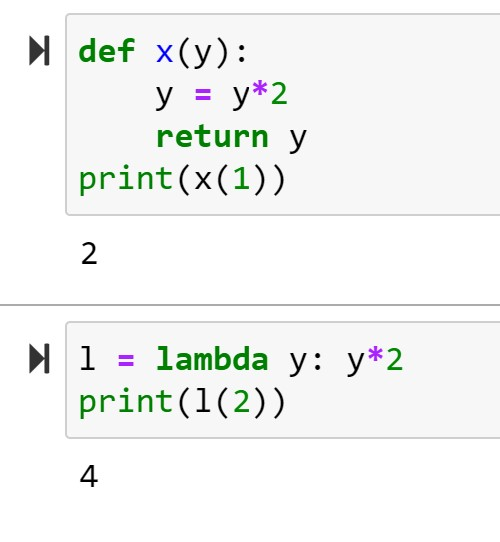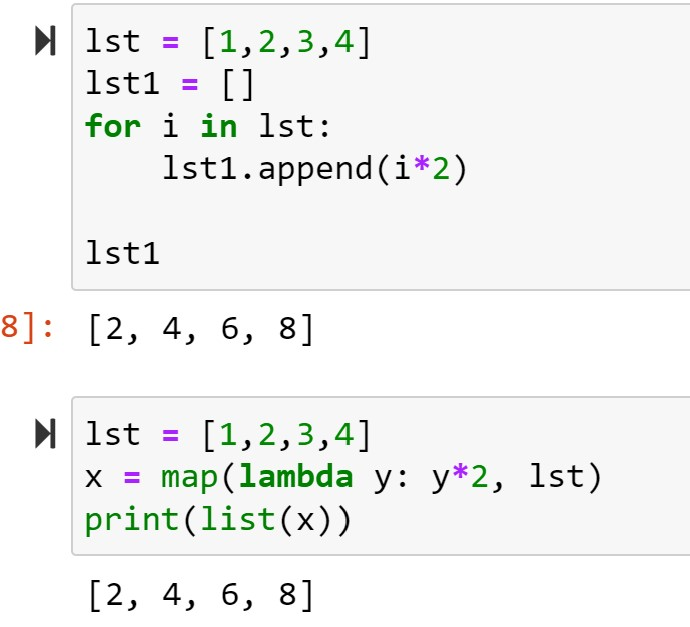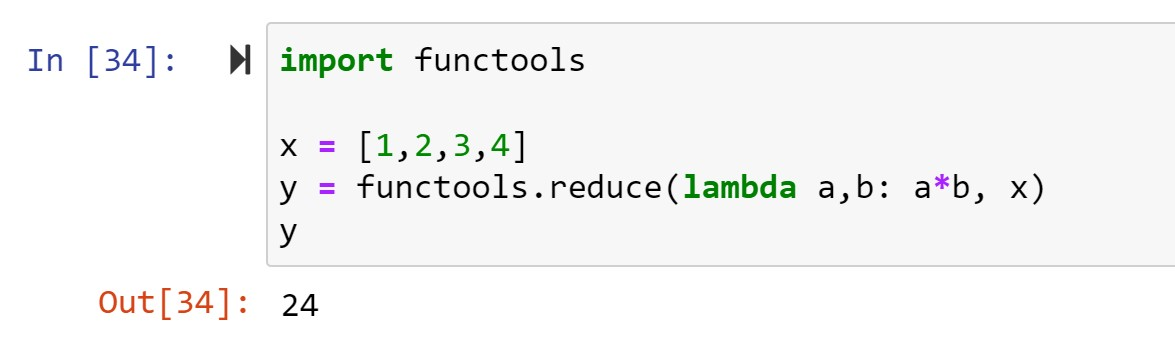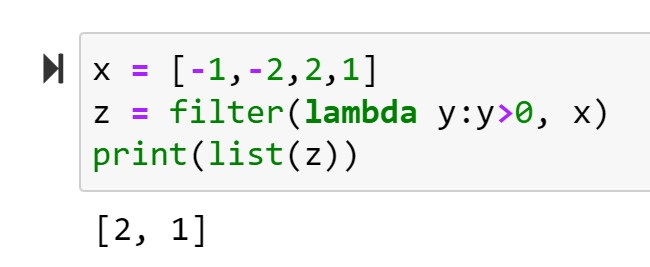# Python: lambda, map(), reduce(), filter()

## Lambda()

One of the guiding principles of Python is simplicity. In fact, you will often see well coded, simplistic Python programs called Pythonic as tribute to the programmer’s talent at crafting clean code.

When I think of Pythonic code, the first thing that comes to my mind is lambda

The best way I can describe lambda is as a short cut function. Look at the function in ln. This function accepts 1 argument “y”.  It then multiplies it by 2 and returns the result.

Now look at ln:

syntax:

x               = lambda            y        :    y*2

ASSIGNED VARIABLE = lambda ARGUMENT: ACTIONTry your code in our online Python console:## map()

Let’s build on lambda and introduce a new function: map()

• lst = [1,2,3,4] – we assign lst a list of numbers
• n = 0 – setting a counter variable
• for i in lst: – start a for loop, iterating through the numbers in lst
• lst[n] = i * 2 – replace each value in lst with the original value * 2
• n += 1 – iterates n by 1
• lst – outputs new values of lstNow let’s examine ln:

• lst = [1,2,3,4] – we assign lst a list of numbers
• x = map(lambda y:y*2, lst) – one at a time, pass each value in lst to the lambda function – multiply these values by 2 and assign the results to x
• x – outputs results

## reduce()

Note: reduce() is now a method of functools module, so you will have to import functools for this to work

Reduce takes all the elements in a list an combines them.

In the example below, x is fed into the lambda function a * b as such:

1*2=2*3=6*4=24Try your code in our online Python console:## filter()

Filter allows you to run a set of values through a function and filter out the false results.

Below we filter out all values from x that are less than 0If you enjoyed this lesson, click LIKE below, or even better, leave me a COMMENT

Last Lesson: Error Handling

Next Lesson: Zip and Unpack

Back to Python Course: Course